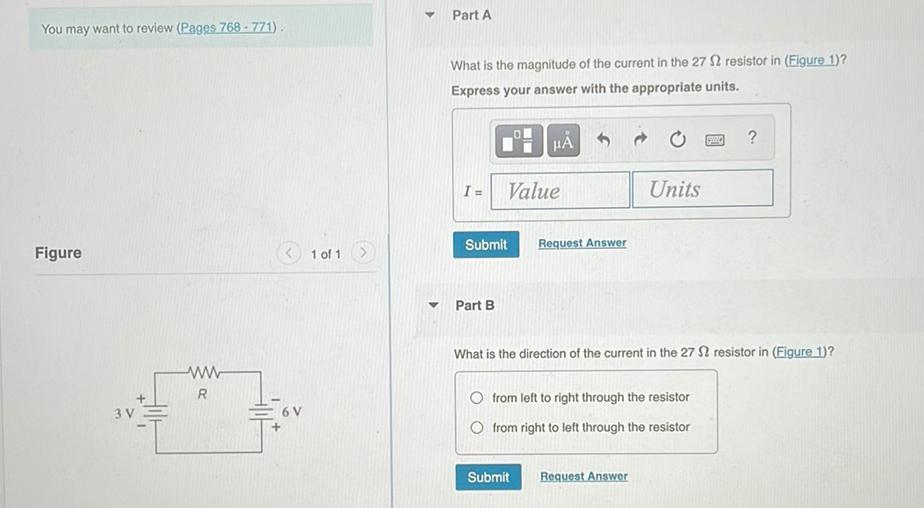Question:

# Part A You may want to review (Pages 768 - 771) What is the magnitude of the current in the 27 12 resistor in (Figure 1)? ExpresPart A You may want to review (Pages 768 - 771) What is the magnitude of the current in the 27 12 resistor in (Figure 1)? Express your answer with the appropriate units. HA ? I= Value Units Submit Request Answer Figure 1 of 1 Part B What is the direction of the current in the 27 12 resistor in (Figure 1)? W R 3 V 6V O from left to right through the resistor Ofrom right to left through the resistor Submit Request Answer